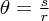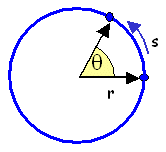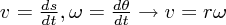## Angular Velocity

• For rotational motion about a point or axis, angular velocity is the rate of change of the angular position with time, or in other words the derivative of the angular position with time:= angular velocity, where θ = the angular position t = time

The direction of the angular velocity vector is perpendicular to the plane of rotation as given by the right-hand rule. The angular velocity is expressed in units of [angular distance/time], often radians per second.

For an object moving in a curved path it can be useful to describe the motion using both angular and linear velocities. Using a fundamental relation for circular geometry:where θ = angles = arc subtended by θ r = radius of the circle

The magnitudes of the linear and angular velocities are related by:where v = linear velocity r = distance from the axis of rotation ω = angular velocity

Note that we are not using vector notation in this expression, rather it is the magnitude of the velocities that follow the relation.